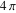# Volume 14 (page 2)

## Perimetric Complexity of Binary Digital Images »NBCDFPDF

### Notes on Calculation and Relation to Visual Complexity

Published February 17, 2012

Perimetric complexity is a measure of the complexity of binary pictures. It is defined as the sum of inside and outside perimeters of the foreground, squared, divided by the foreground area, divided by. Difficulties arise when this definition is applied to digital images composed of binary pixels. In this article we identify these problems and propose solutions. Perimetric complexity is often used as a measure of visual complexity, in which case it should take into account the limited resolution of the visual system. We propose a measure of visual perimetric complexity that meets this requirement. Read More »

## Motion of a Spinning Top »NBCDFPDF

Published February 16, 2012

Both approximate and exact solutions for the motion of a spinning top are constructed with the help of quaternions. Read More »

## Evaluation of Gaussian Molecular Integrals »NBCDFPDF

### I. Overlap Integrals

Published February 16, 2012

This article discusses the evaluation of molecular overlap integrals for Gaussian-type functions with arbitrary angular dependence. As an example, we calculate the overlap matrix for the water molecule in the STO-3G basis set. Read More »

## Exploring Reflection and Transmission Coefficients in Elastic Media »NBCDFPDF

Published January 26, 2012

Reflection and transmission (scattering) of plane waves at a planar boundary between two elastic half-spaces are important fundamental processes in seismology. Such plane waves may be compressional (P) or shear (S) in an elastic medium. In this article we apply Mathematica to computing the complex algebraic expressions describing the reflection and transmission amplitudes, phases, and angles of propagation. I also illustrate the energy flux quantities. Brewster’s angle, which represents where zero reflected energy occurs, is an important variable for SH waves, which are polarized out of the cross section, and is dependent on both the velocity and density ratio of the two half-spaces. For the P-SV system, the P and SV waves are polarized in the plane of the cross section. Poisson’s ratio (a function of the P and S velocities) affects the energy flux behavior in the case of incident SV but not incident P. Also for the P-SV system, there are four critical angles that affect the energy flux behavior. I also study the limiting P-SV case where the top medium becomes a vacuum, thus creating a “free surface.” For the P-SV system, energy flux of the four scattered waves for any incident wave can be partitioned into top and bottom layer contributions and into total P and total SV contributions, providing further insight into the nature of P-SV scattering. The single-boundary formulation can easily be extended to a stack of layers giving the amplitude and phase at receivers offset along the surface from the source. Read More »

## Mathematical Exploration of Kirkwood Gaps »NBCDFPDF

Published January 16, 2012

We first solve the planar Kepler problem of an asteroid’s motion, perturbed by the gravitational pull of Jupiter. Analyzing the resulting differential equations for its orbital elements, we demonstrate the mechanism for creating a gap at the 2:1 resonance (the asteroid making two orbits for Jupiter’s one), and briefly mention the case of other resonances (3:2, 3:1, etc.). We also discuss reasons why the motion becomes chaotic at these resonances. Read More »